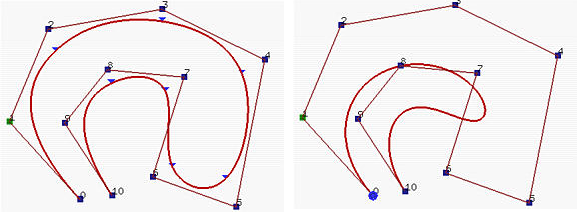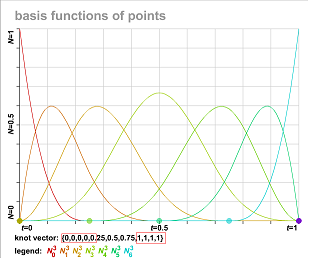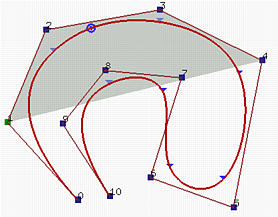# B-Spline(三)样条曲线的性质

1.定义:

1. B样条 的控制点个数与曲线次数无关，因此B样条的自由度更大，可以定义很多控制点又不用担心曲线次数过高而计算困难。
2. B样条有多个节点区间，使得其为分段函数，而Bezier曲线只有一个区间。

1. B样条上任意一个区间$$[u_i,u_{i+1})$$上的曲线的定义都是一个Bezier。因此可以将B样条转为分段bezier曲线。
2. Bezier是一种特殊的样条曲线。后面会详细分析。

2.性质

1. B样条上任意一个区间$$[u_i,u_{i+1})$$上的曲线的定义都是一个Bezier曲线。相对于bezier曲线，B样条曲线能够更好的控制曲线形状。如下图，左侧是11个控制点构成的3次样条曲线，右侧是同样控制点构成的10次Bezier曲线，其曲线可控制性要比样条曲线差。如果定义一样的控制点，阶次越低，曲线离控制点就越远。当然了，如果曲线的阶次为1，那么曲线就是控制多边形本身==!。2. m,n,p必须要满足的关系是m=n+p+1。这个在基函数的定义里面就证明了。
3. “clamped”B样条（如上图左），就是首尾节点重复度分别为p+1的样条，曲线的首尾点分别和首尾控制点相同。这个和bezier曲线一样。如何证明呢？可以将u=0带入de-Boor迭代式中求解，只不过，因为重复节点(0长度区间)的存在，我们需要定义$$\frac{0}{0}=0$$。实际上，可以通过上节我介绍的swf文件直接观察B样条基函数的情况。如下图，$$N_0,N_6$$在节点区间首末点处均为1，其他基函数为0，那么带入B样条，可以得出$$C(0)=P_0;C(1)=P_6$$。4. 强凸包性:对应区间$$[u_i,u_{i+1})$$上曲线一定在由p+1个控制点$$P_{i-p},…,P{i}$$构成的凸包之中。因为在这段区间上，$$N_{i-p},…,N{i}$$是大于0的（原文为非0），而且根据基函数的性质，几者相加等于1，那么中间的曲线相当于这几个点的“加权平均”，自然位于其凸包之内。所谓强凸包性，指的是曲线要比凸包要小的多。这个性质在曲线的折线化（离散）时非常有用。如果我们能让控制点构成直线，那么对应的曲线也能形成直线。5. 局部修改模式：修改控制点$$P_i$$，那么其影响的曲线范围仅限于区间$$[u_i,u_{i+p+1}$$。对应的是基函数的非零范围。因此，大家可以记住，$$N_i$$与$$P_i$$是一对，如果$$N_i$$非零，那么$$P_i$$的变化就会对对应区间产生影响。在实际生产中，用户通常通过加入节点，增加节点个数的方式获取更多的控制点，进而利用B样条的局部修改模式细化调整曲线，而不影响曲线整体走势。
6. 样条曲线在节点处的连续性为$$C^p$$，在重复度为k的节点处的连续性为$$C^{p-k}$$。普通节点的重复度为1。因为$$C^2$$已经能够保证曲率连续了，所以在一般的业务应用中，三次(cubic)样条曲线最为常用。
7. 变差减少性(Variation Diminishing Property)：这条性质与Beizier曲线的相近，如果一条直线与样条曲线的控制多边形的交点数为N,那么这条直线与样条曲线的交点数应该不大于N。
8. Bezier曲线是特殊的样条曲线。因为Bezier曲线的控制点个数为次数+1，所以，当将样条曲线的控制点取p+1个，节点序列取$$\{0,0,…,0,1,1,…1\}$$曲线即为bezier曲线。
9. 仿射不变性：这一条的意思是，如果需要对样条曲线做变换，那么只需要对其控制点序列做变换就可以了。其参数区间，次数保持不变。非常好的性质。

补充，当$$u=u_i$$时，$$N_i=0$$。而且对应区间上至多有p+1个非零基函数。因此性质第4条强凸包性应该是在区间$$(u_i,u_{i+1})$$且p<i<m-p时成立。

B样条的优点：

## 《B-Spline(三)样条曲线的性质》上有3个想法

1.Rocket说道：

想请问一下，三阶的三个点的b样条曲线，能不能举一个u的节点的例子？这样的曲线怎么设置节点，才可以变成bezier曲线？

•Rocket说道：

因为按照m=n+p+1，三个控制点，三阶b样条，两个端点分别需要4个节点，但是公式算出来m=7不满足要求，是不是就不能存在？

•whudj说道：

我觉得可以做一个一般性的理解，比如说对于一阶的“曲线”也即直线，需要2个点（至少）确定； 对于二阶曲线，比如抛物线，需要3个点（至少）来确定；对于三阶曲线，是需要4个点（至少）来确定……
所以说，3个点是无法插值出三阶Bazier曲线的；需要至少4个点来确定一条三阶Bazier曲线；